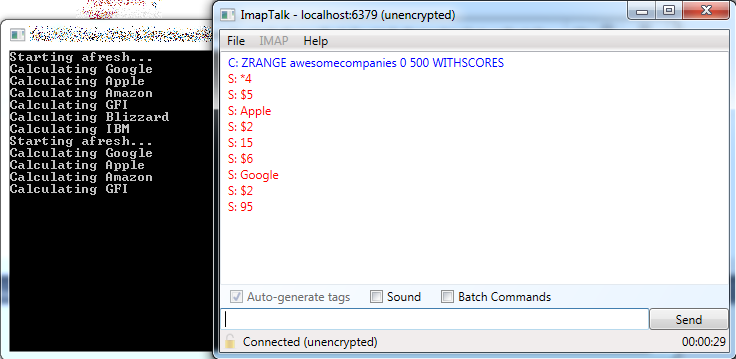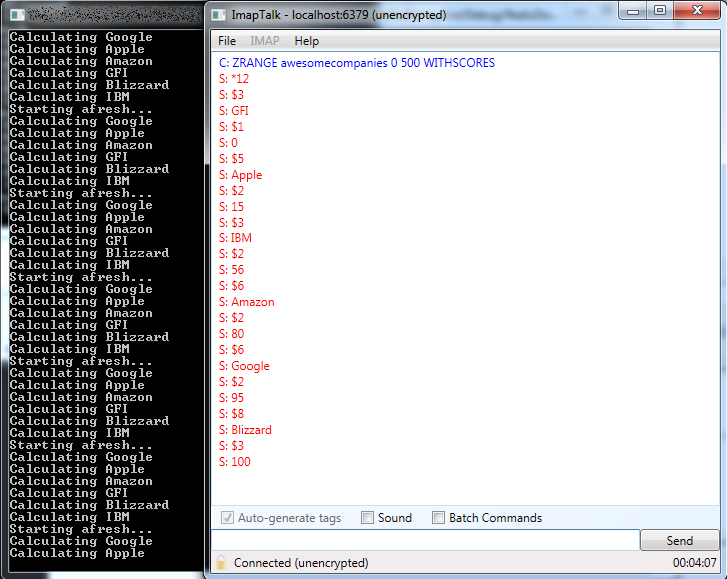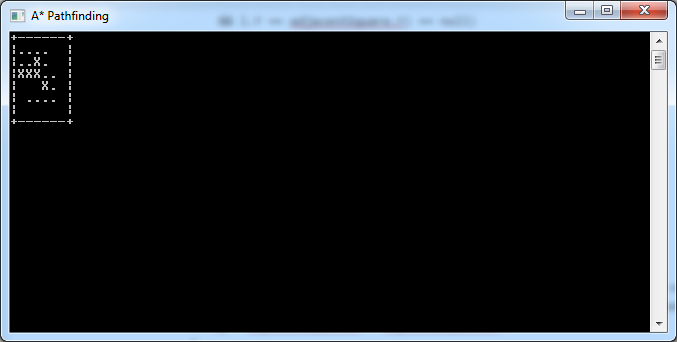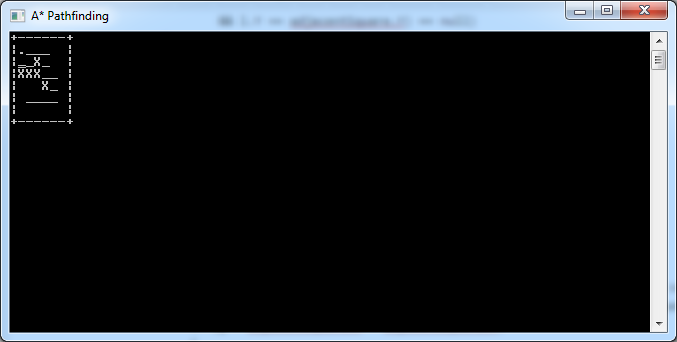# Double Buffering in Redis

This article deals with a specific situation. You have a program that is continuously building a data structure from scratch. This data structure takes long (i.e. several seconds) to build, but is continuously accessed by clients. While it is being built, clients will end up retrieving partial data. This article explains how a well-known technique from computer graphics can be used in Redis to address this problem. The full source code is available at BitBucket.

# The Problem

Like just about anything, this scenario is best demonstrated with an example. So we’ll first start with some code to set up a client connection to Redis:

```        private static Lazy<ConnectionMultiplexer> LazyConnection
= new Lazy<ConnectionMultiplexer>(() =>
{
var config = new ConfigurationOptions();
config.AbortOnConnectFail = false;

return ConnectionMultiplexer.Connect(config);
}
);

public static ConnectionMultiplexer Connection
{
get
{
return LazyConnection.Value;
}
}
```

The code above is based on the feedback I received from this StackOverflow question and is the proper way to get a connection set up using StackExchange.Redis. You’ll need to install the StackExchange.Redis package via NuGet before you can use this code.

Our actual program code is extremely simple. We establish a connection (via the property above) and then continuously call a method that populates our data structure:

```        static void Main(string[] args)
{
var connection = Connection;
var database = connection.GetDatabase(0);

while (true)
PopulateAwesomeCompanies(database);
}
```

We use this infinite loop because the nature of the data may be dynamic, for example items may be removed, and it may be hard to keep track of them. So the data structure is simply rebuilt regularly.

For this simple example, our data structure is going to be a Redis sorted set that stores the most awesome companies to work for, sorted by awesomeness. Here is the data we’re going to be using:

```        private static string[] companies = new string[]
{
"Apple",
"Amazon",
"GFI",
"Blizzard",
"IBM"
};

private static int[] companyScores = new int[] { 95, 15, 80, 0, 100, 56 };
```

The method that builds the data structure is also very simple. It starts off by erasing the existing data, and then for each company, it calculates their awesomeness (simulated by a simple delay) and adds them to the sorted set:

```        public static void PopulateAwesomeCompanies(IDatabase database)
{
var key = "awesomecompanies";

Console.WriteLine("Starting afresh...");

for (int i = 0; i < 6; i++)
{
Console.WriteLine("Calculating {0}", companies[i]);

// simulate expensive computation

// add company with respective score
}
}
```

The problem with this approach is that anytime a client accesses this data structure while it’s still being built, they will get partial data:That sucks, because we want the data to be fresh, but we also want it to be complete when it is handed to clients.

# Double Buffering in Computer Graphics

There is a technique in computer graphics called double buffering which solves a similar problem. Here’s some background from Wikipedia:

“In computer graphics, double buffering is a technique for drawing graphics that shows no (or less) flicker, tearing, and other artifacts.

“It is difficult for a program to draw a display so that pixels do not change more than once. For instance to update a page of text it is much easier to clear the entire page and then draw the letters than to somehow erase all the pixels that are not in both the old and new letters. However, this intermediate image is seen by the user as flickering. In addition computer monitors constantly redraw the visible video page (at around 60 times a second), so even a perfect update may be visible momentarily as a horizontal divider between the “new” image and the un-redrawn “old” image, known as tearing.

“A software implementation of double buffering has all drawing operations store their results in some region of system RAM; any such region is often called a “back buffer”. When all drawing operations are considered complete, the whole region (or only the changed portion) is copied into the video RAM (the “front buffer”); this copying is usually synchronized with the monitor’s raster beam in order to avoid tearing. Double buffering necessarily requires more memory and CPU time than single buffering because of the system memory allocated for the back buffer, the time for the copy operation, and the time waiting for synchronization.”

In short, double buffering involves the following steps:

1. Draw the next frame (screen image) in a temporary location in memory that isn’t directly visible to the user (the back buffer)
2. Swap the front buffer (the actual image on the screen) with the back buffer

That way, the change between the old image and the new one is instantaneous and the user will never notice the difference.

# Double Buffering in Redis

We can apply a similar technique in Redis as follows:

1. Build the new sorted set using a separate, temporary key
2. Rename the temporary key to the existing key (implicitly deletes the temporary key)

This is how it works out in practice:

```        public static void PopulateAwesomeCompanies(IDatabase database)
{
var tempKey = "awesomecompaniestemp";
var key = "awesomecompanies";

Console.WriteLine("Starting afresh...");

for (int i = 0; i < 6; i++)
{
Console.WriteLine("Calculating {0}", companies[i]);

// simulate expensive computation

// add company with respective score
}

// replace actual data in key with fresh data from tempKey
database.KeyRename(tempKey, key);
}
```

Here, we aren’t deleting any data structure before we begin, as we did before. Instead, we build our fresh sorted set using the temp key, and then copy it over to the actual key (the one that clients will be requesting). We do this using the Redis RENAME command, which copies the data from the temp key to the actual key, and implicitly also deletes the temp key. Since Redis is single-threaded, this operation is atomic and there is no race condition risk while the rename operation is happening.

The result of this is that the client will always have access to the latest complete data, even while the data structure is being rebuilt:# A* Pathfinding Example in C#

The A* pathfinding algorithm is one of the most popular ways of computing the shortest path between two points for game development. “Introduction to A* Pathfinding” by Johann Fradj illustrates the algorithm pretty nicely. I’ve written a C# implementation based on his example code, intended as nothing more than a learning exercise, and I am sharing it in this article. You can get the full source code directly from BitBucket; if you run it, you’ll see the algorithm run step by step.

Our A* algorithm will compute the shortest path between two points, A and B, on a small square grid of tiles. In order to really see it at work, we’ll introduce some obstacles, marked as X in the map below:

```            string[] map = new string[]
{
"+------+",
"|      |",
"|A X   |",
"|XXX   |",
"|   X  |",
"| B    |",
"|      |",
"+------+",
};
```

The A* algorithm needs to keep track of the tiles it’s visited (closed list) and the ones it’s considering (open list). For this we define a Location class:

```    class Location
{
public int X;
public int Y;
public int F;
public int G;
public int H;
public Location Parent;
}
```

Aside from the tile coordinates, each visited tile has three scores (F, G and H). The G score is the distance from the starting point, and the H score is the estimated distance from the destination (calculated as the city block distance). The F score is just G + H. For a full explanation of these values, read the reference article, “Introduction to A* Pathfinding“.

The H score is very simple to calculate – it’s just the horizontal and vertical distance from the current tile to the destination (ignoring any obstacles):

```        static int ComputeHScore(int x, int y, int targetX, int targetY)
{
return Math.Abs(targetX - x) + Math.Abs(targetY - y);
}
```

The Location class also has a Parent property. That’s used to store the previous tile, and it is needed to keep track of the path itself by the time the destination is reached.

Before we start the algorithm, we initialise some values:

```            Location current = null;
var start = new Location { X = 1, Y = 2 };
var target = new Location { X = 2, Y = 5 };
var openList = new List<Location>();
var closedList = new List<Location>();
int g = 0;

// start by adding the original position to the open list
```

The g value is the G-score; remember it’s the distance from the starting location to the current tile. We keep track of this simply by incrementing its value each time we move to a new tile.

We add the starting location to the open list, so that the algorithm can begin working. The algorithm itself runs in a loop:

```            while (openList.Count > 0)
{
// algorithm's logic goes here
}
```

For each iteration, the A* algorithm will retrieve the tile with the lowest F-score:

```                // get the square with the lowest F score
var lowest = openList.Min(l => l.F);
current = openList.First(l => l.F == lowest);
```

This is not necessarily the most efficient way of doing this, but works pretty well for this example. Also note that if there are multiple locations with the same F-score, it doesn’t really matter which is chosen (here we just take the first one) – again see “Introduction to A* Pathfinding” for details.

```                // add the current square to the closed list

// remove it from the open list
openList.Remove(current);
```

Here we add the current tile to the closed list, and remove it from the open list, to prevent it from being revisited in a subsequent iteration.

```                // show current square on the map
Console.SetCursorPosition(current.X, current.Y);
Console.Write('.');
Console.SetCursorPosition(current.X, current.Y);
```

The code above is not part of the A* algorithm. It simply marks the current tile with a dot and waits for a second so that you can follow the algorithm’s progress interactively.

```                // if we added the destination to the closed list, we've found a path
if (closedList.FirstOrDefault(l => l.X == target.X && l.Y == target.Y) != null)
break;
```

There are two conditions that allow the A* algorithm to terminate. One is that there are no more tiles in the open list to process, which would indicate that there is no path between A and B (we aren’t catering for this scenario in this example). The other is when the path is actually found, and that is catered for by the code above.

The rest of the algorithm evaluates adjacent tiles (i.e. the ones immediately one tile left, right, up and down from the current one):

```                var adjacentSquares = GetWalkableAdjacentSquares(current.X, current.Y, map);
g++;
```

```        static List<Location> GetWalkableAdjacentSquares(int x, int y, string[] map)
{
var proposedLocations = new List<Location>()
{
new Location { X = x, Y = y - 1 },
new Location { X = x, Y = y + 1 },
new Location { X = x - 1, Y = y },
new Location { X = x + 1, Y = y },
};

return proposedLocations.Where(
l => map[l.Y][l.X] == ' ' || map[l.Y][l.X] == 'B').ToList();
}
```

So back in the algorithm’s loop, we then have a loop over adjacent squares, computing their scores and adding them to the open list when it makes sense to do so:

```                foreach(var adjacentSquare in adjacentSquares)
{
// if this adjacent square is already in the closed list, ignore it
if (closedList.FirstOrDefault(l => l.X == adjacentSquare.X
&& l.Y == adjacentSquare.Y) != null)
continue;

// if it's not in the open list...
if (openList.FirstOrDefault(l => l.X == adjacentSquare.X
&& l.Y == adjacentSquare.Y) == null)
{
// compute its score, set the parent

// and add it to the open list
}
else
{
// test if using the current G score makes the adjacent square's F score
// lower, if yes update the parent because it means it's a better path
{
}
}
}
```

There’s some duplicate code in the if/else statement above, but I preferred to leave it that way as it’s more readable (which is more important here), especially if you’re following along with “Introduction to A* Pathfinding“.

That’s the last thing in the algorithm itself. So once it’s done, you can see the locations that were examined thanks to the dots drawn on the map:When it’s done, the program will run the following code, tracing its way back from destination to start by following the Parent property:

```            // assume path was found; let's show it
while (current != null)
{
Console.SetCursorPosition(current.X, current.Y);
Console.Write('_');
Console.SetCursorPosition(current.X, current.Y);
current = current.Parent;